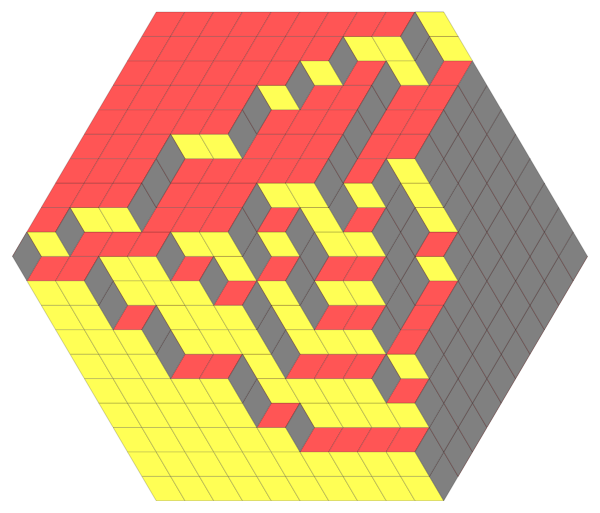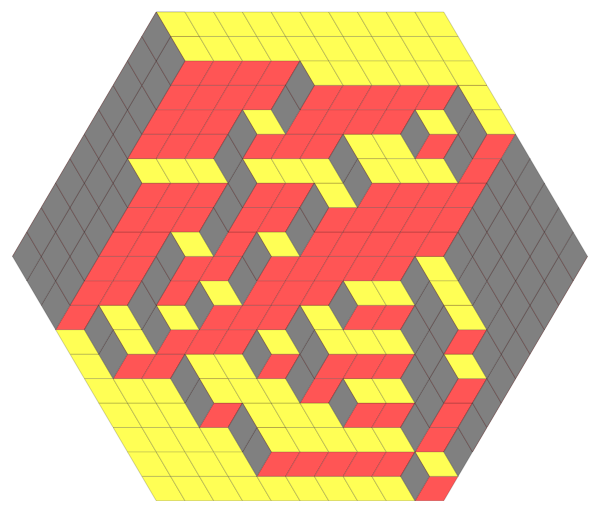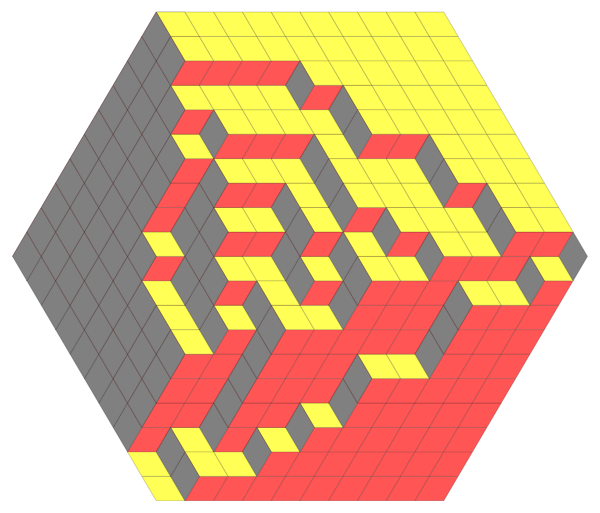# Mirroring deterministic dynamics on q-vol lozenge tilings inverting the parameter q

#### Leonid Petrov

Continuing with the setup of the previous simulation (see here), we consider a simple, deterministic transformation of the tiling, when at each step on one of the levels the vertical lozenges are mirrored with respect to the middle of the segment [min,max] to which each of them belong. The dynamics starts from an exact sample of the measure $q^{-volume}$ (which is produced by Vadim Gorin’s program ). Then, by mirroring vetical lozenges to the left, the measure $q^{-volume}$ becomes the measure $q^{volume}$. At each step, the distribution of the tiling is the same as in the previous simulation with random moves. However, under the mirroring dynamics the vertical tiles can move both left and right, and the Markovian nature of the behavior on the left edge is lost.

Here are three states of the tiling in the beginning, the middle of the simulation, and the end. We see that the beginning and the ending configuration are exactly the same, up to reflection about the center of the hexagon.### Data file format

The data file is a list of lists of lists in Mathematica-readable format, of the form $$\{ \lambda(1),\lambda(2),\ldots,\lambda(T) \},$$ where each $\lambda(t)$ is a list of weakly interlacing integer coordinates of the form $$\{ \{ 47 \},\{ 50,47 \} , \{ 50,49,47 \} ,\ldots, \} .$$ Here $t$ is the time variable. The simulation data can be “coarse” in larger tilings, or finer with every step of the Markov chain recorded.

## code • (Main GitHub repo)

(note: parameters in the code might differ from the ones in simulation results below)

## simulation results

1. ##### Movie, size 10 • (data: 100 KB) • (graphics: 500 KB)
Hexagon $10\times 10\times 10$, $q=0.85$.## references

1. L. Petrov, A. Saenz. In preparation (2019)
2. A.Borodin, V. Gorin. Shuffling algorithm for boxed plane partitions. Advances in Mathematics, 220 (6) (2009). 1739-1770, • https://arxiv.org/abs/0804.3071https://www.sciencedirect.com/science/article/pii/S0001870808003253
3. A. Bufetov, L. Petrov. Yang-Baxter field for spin Hall-Littlewood symmetric functions (2017) • https://arxiv.org/abs/1712.04584